# Strong matchings and covers

 Importance: High ✭✭✭
 Author(s): Aharoni, Ron
 Subject: Graph Theory » Infinite Graphs
 Keywords: cover infinite graph matching
 Posted by: mdevos on: October 23rd, 2007

Letbe a hypergraph. A strongly maximal matching is a matching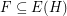so that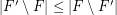for every matching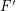. A strongly minimal cover is a (vertex) coverso that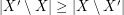for every cover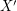.

Conjecture   Ifis a (possibly infinite) hypergraph in which all edges have size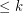for some integer, thenhas a strongly maximal matching and a strongly minimal cover.

The theory of matching in finite graphs is quite well understood. Now, thanks to the work of Aharoni and others, much of this theory has been extended to infinite graphs. On the other hand, matching in hypergraphs - both finite and infinite - is a subject where our knowledge apears to be lacking. The above conjecture asserts a rather basic property of hypergraphs which would be nice to verify.

This conjecture is (of course) trivial for finite hypergraphs, but it looks very difficult for infinite ones. It has been proved by Aharoni [A2] for the case when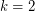, that is, for infinite graphs. Here the key tool is an infinite version of the Tutte-Edmonds-Gallai decomposition theorem [A1].

Next we offer another interesting conjecture of Aharoni on minimal covers.

Conjecture   Ifis a (possibly infinite) graph andis the hypergraph of independent sets in, thenhas a strongly minimal cover.

## Bibliography

[A1] R. Aharoni, Matchings in infinite graphs. J. Combin. Theory Ser. B 44 (1988), no. 1, 87--125. MathSciNet.

*[A2] R. Aharoni, Infinite matching theory. Directions in infinite graph theory and combinatorics (Cambridge, 1989). Discrete Math. 95 (1991), no. 1-3, 5--22. MathSciNet.

* indicates original appearance(s) of problem.